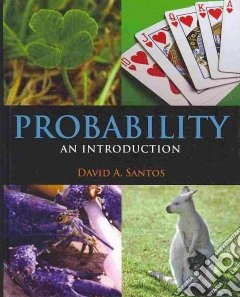ricerca
avanzata

# Probability

## Un libro in lingua di Santos David A. edito da Jones & Bartlett Learning, 2010

• € 106,60
• Il prezzo è variabile in funzione del cambio della valuta d’origineThis book/CD-ROM introductory text on probability requires minimal algebraic skills and features examples and solved exercises from various fields. It can be used for a discrete probability course and for an introduction to calculus-based probability. It will also be useful to students in need of probability training for mathematical competitions and for those training for the SOA/CAS Exam P actuarial exams. The text begins with sets and functions, then covers combinatorics, probability, conditional probability, discrete random variables, and densities. Reference appendices of equations are included. In order to make the text accessible to non-calculus trained students, most of the examples, results, and theorems don't use calculus, with calculus-based material relegated to the final chapter. For more advanced students, there are classical problem sections in each chapter and computer explorations using Maple. Maple is used as a calculator throughout the book. The CD-ROM contains computer code and project files. Information on the author is not given. Annotation ©2010 Book News, Inc., Portland, OR (booknews.com)